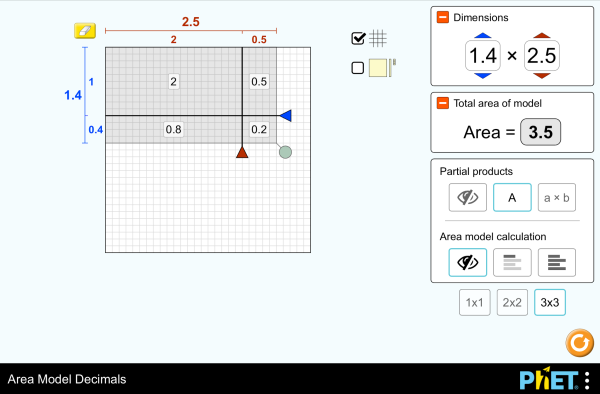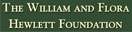# Pinta-alamalli desimaaleillaLataaUpota sulje Upota tämän simulaatio toiselle verkkosivulle Käytä tätä HTML-koodia upottaaksesi simulaation haluamallesi verkkosivulle. Voit muuttaa simulaation leveyttä ja korkeutta muuttamalla koodin "width" ja "height" attribuutteja. Upota kuva, joka käynnistää simulaation sitä klikatessa.
Käynnistä klikkaamalla
Käytä tätä HTML-koodia näyttääksesi kuvakaappauksen, jossa lukee "Käynnistä klikkaamalla". Kertolasku Decimals Area Model PhET-projektia tukevatja opettajat kuten sinä.

### Sisältö

• Decimals
• Area Model
• Factors
• Partial Products

### Description

Build rectangles of various sizes and relate multiplication to area. Partition a rectangle into smaller areas and discover new strategies for multiplying decimals!

### Esimerkkejä oppimistavoitteista

• Recognize that area represents the product of two numbers and is additive.
• Develop and justify a strategy that uses the area model to simplify a decimal multiplication problem.
• Devise a strategy to determine the product of two rational numbers (or an integer and a rational number) by representing the product as an area or the sum of areas.
• Use an area model to understand why multiplying a given number by a decimal less than 1 results in a product smaller than the given number.
• Interpret the product of two rational numbers using base-ten tiles

### Standards Alignment

#### Common Core - Math

5.NF.B.4
Apply and extend previous understandings of multiplication to multiply a fraction or whole number by a fraction.
5.NF.B.4a
Interpret the product (a/b) × q as a parts of a partition of q into b equal parts; equivalently, as the result of a sequence of operations a × q ÷ b. For example, use a visual fraction model to show (2/3) × 4 = 8/3, and create a story context for this equation. Do the same with (2/3) × (4/5) = 8/15. (In general, (a/b) × (c/d) = ac/bd.)
5.NF.B.4b
Find the area of a rectangle with fractional side lengths by tiling it with unit squares of the appropriate unit fraction side lengths, and show that the area is the same as would be found by multiplying the side lengths. Multiply fractional side lengths to find areas of rectangles, and represent fraction products as rectangular areas.
5.NF.B.5
Interpret multiplication as scaling (resizing), by:
5.NF.B.5a
Comparing the size of a product to the size of one factor on the basis of the size of the other factor, without performing the indicated multiplication.
5.NF.B.5b
Explaining why multiplying a given number by a fraction greater than 1 results in a product greater than the given number (recognizing multiplication by whole numbers greater than 1 as a familiar case); explaining why multiplying a given number by a fraction less than 1 results in a product smaller than the given number; and relating the principle of fraction equivalence a/b = (n × a)/(n × b) to the effect of multiplying a/b by 1.
Version 1.0.9

### Teacher-Submitted Activities

Area Model Decimals - Grade 6Maura Dudley YK Guided Matematiikka
Arabia All العربيةنموذج مساحة الأعداد العشرية
Baski All BasqueAzalera-modeloa hamartarrekin
Bosnia All BosanskiMatematički model površina - Decimalni brojevi
Brasilian portugali All português (Brasil)Modelo de Área: Decimais
Englanti All EnglishArea Model Decimals
Espanja All españolModelo de Áreas: Decimales
Espanja (Meksiko) All español (México)Modelo de Áreas: Decimales
Espanja (Peru) All español (Perú)Modelo de área con decimales
Gudžarati All Gujaratiઅપૂર્ણાંકમાં ક્ષેત્રફળનું મોડેલ - નિકુંજ સવાણી દ્વારા
Hindi All हिंदीनमूने का क्षेत्रफल - दाशमिक
Hollanti All NederlandsOppervlakte model decimalen
Italia All italianoRappresentazione grafica del prodotto di numeri decimali
Japani All 日本語面積と式の展開（小数）
Kazakki All KazakhАуданды ондық бөлшектермен есептеу
Kiina - perinteinen All 中文 (台灣)Area Model Decimals_面積模型與小數
Kiina - yksinkertaistettu All 中文 (中国)面积模型：小数
Korea All 한국어면적 모형: 소수
Kreikka All ΕλληνικάΥπολογισμός εμβαδού - Δεκαδικοί
Latvia All LatviešuLaukuma modeļa decimāldaļskaitļi
Mongoli All Монгол (Монгол)Талбайн Загвар Бутархайгаар
Puola All polskiModel pola - ułamki dziesiętne
Ranska All françaisModélisation par les aires avec décimales
Ruotsi All svenskaAreamodellen och decimaltal
Saksa All DeutschFlächenmodell Dezimalzahlen
Serbia All СрпскиМатематика преко површина-Децимални бројеви
Tamili All Tamilமாதிரிகளின் தசமப் பரப்பளவு
Tanska All DanskArealmodel, decimaler
Unkari All magyarTerületmodell: tizedesjegyek
Venäjä All русскийВычисление площади с десятичными дробями
Vietnam All Tiếng ViệtSố thập phân với mô hình diện tích

The new HTML5 sims can run on iPads and Chromebooks, as well as PC, Mac, and Linux systems.

iOS 11+ Safari

Android:
Not officially supported. If you are using the HTML5 sims on Android, we recommend using the latest version of Google Chrome.

Chromebook:
The HTML5 and Flash PhET sims are supported on all Chromebooks.
Chromebook compatible sims

Windows Systems:

Macintosh Systems:
macOS 10.9.5+, Safari 9+, latest version of Chrome.

Linux Systems:

Suunnitteluryhmä Kolmannen osapuolen kirjastot Kiitokset
• Amy Hanson (lead designer)
• Amanda McGarry (co-lead)
• Jonathan Olson (developer)
• Karina Hensberry
• Susan Miller
• Ariel Paul
• Kathy Perkins
• almond-0.2.9.js
• base64-js-1.2.0.js
• FileSaver-b8054a2.js
• font-awesome-4.5.0
• game-up-camera-1.0.0.js
• himalaya-0.2.7.js
• jama-1.0.2
• jquery-2.1.0.js
• lodash-4.17.4.js
• pegjs-0.7.0.js
• seedrandom-2.4.2.js
• text-2.0.12.js
• TextEncoderLite-3c9f6f0.js